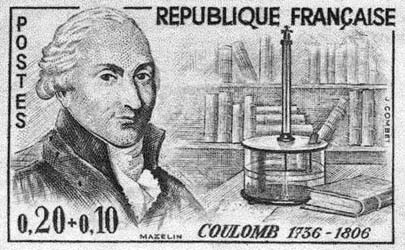This tiny post is about the physical origin of a term used by mathematicians in analysis, probability, and geometry. If ${(X_t)}_{t\geq0}$ is a continuous time Markov process with state space $E$ and infinitesimal generator $L$ then its carré du champ operator is defined for any $f:E\to\mathbb{R}$ by

$$\Gamma f=\frac{1}{2}(L(f^2)-2fLf).$$ It appears naturally in the analysis of the conditional laws of the Markov process. Namely, denoting ${(P_t)}_{t\geq0}={(\mathrm{e}^{tL})}_{t\geq0}$ the Markov semigroup, we get, informally, for any $f$ and $t\geq0$,
$$\mathrm{Var}_{P_t}(f)=P_t(f^2)-P_t(f)^2=(f^2+tL(f^2)+\cdots)-(f+tLf+\cdots)^2=2t\Gamma(f)+o(t)$$ (and the higher order terms lead to the more general notion of $\Gamma_n$). In the same spirit,
$$\mathrm{Var}_{P_t}(f)=P_t(f^2)-P_t(f)^2=\int_0^t\!\partial_sP_s(P_{t-s}(f)^2)\,\mathrm{d}s=2\int_0^t\! P_s(\Gamma(P_{t-s}(f)))\,\mathrm{d}s,$$

where we used the following formula, denoting $G_s=g^2$ and $g=P_{t-s}(f)$ and using the fact that $F(s,u)=P_s(u)$ is linear with respect to the second variable,

\begin{align}\partial_sP_s(P_{t-s}(f)^2)&=\partial_s(F(s,G_s))\\ &=(\partial_1F)(s,G_s)+(\partial_2F)(s,G_s)\partial_sG_s\\ &=P_s(L(g^2))-F(s,\partial G_s)\\ &=P_s(L(g^2)-gLg)\\ &=2P_s(\Gamma P_{t-s}f). \end{align}

In the basic example of the standard Brownian Motion on $E=\mathbb{R}^d$, we have $L=\frac{1}{2}\Delta$, $P_t(f)(x)=\mathbb{E}(X_t\mid X_0=x)=\mathbb{E}(f(x+\sqrt{t}G))$, $G\sim\mathcal{N}(0,I_d)$, and we get

$$\Gamma(f)=\frac{\Delta(f^2)-2f\Delta f}{2}=\frac{(\partial_1f)^2+\cdots+(\partial_df)^2}{2}=\frac{1}{2}\left\Vert\nabla f\right\Vert^2.$$ The name carré du champ comes from the mathematical modeling of electrostatics. Namely if $\mu$ is a distribution of charges in $\mathbb{R}^d$ then the Coulomb potential at point $x\in\mathbb{R}^d$ is given by

$$U^\mu(x)=(\mu*g)(x)=\int g(x-y)\mu(\mathrm{d}y)$$ where $*$ is the convolution and $g$ is the Coulomb (or Newton) kernel given for $d\geq2$ by

$$g(x)=c_2\mathbf{1}_{d=2}\log\frac{1}{|x|}+c_3\mathbf{1}_{d\geq3}\frac{1}{{\left\Vert x\right\Vert}^{d-2}}.$$The Coulomb electric force generated by the distribution $\mu$ at point $x$ for a unit charge is $\nabla U^\mu(x)$. Also the quantity $\left\Vert\nabla U^\mu\right\Vert^2$ is the square of the (norm) of the (electric) field: carré du champ in French, see .

The electrostatic energy of self-interaction of this distribution of charges is given by

$$\mathcal{E}(\mu)=\int\!U^\mu(x)\mu(\mathrm{d}x).$$ Since $g$ is the fundamental solution of the Laplace equation we have $\Delta g=-c_d\delta_0$ in distributional sense and thus, by an integration by parts

$$\mathcal{E}(\mu)=-c_d\int\!U^\mu(x)\Delta U^\mu(x)\mathrm{d}x=c_d\int\!\left\Vert\nabla U^\mu(x)\right\Vert^2\mathrm{d}x.$$ The carré du champ plays a central role for the analysis and geometry of Markov diffusion operators in the Bakry-Émery theory, see . The electrostatic Coulomb energy is a central concept in potential theory. The electrostatic Coulomb energy plays also a central role in the asymptotic analysis of Coulomb gases, notably those emerging from Random Matrix Theory, see for instance the modest contribution  and references therein. The funny thing is that most specialists of the analysis and geometry of Markov diffusion operators are not aware of the Coulomb physical (hi)story about their carré du champ!